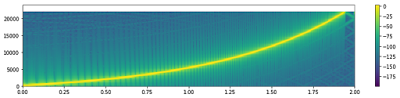Sunday, May 7, 2017

NNResample package on PyPI

In the previous post, I described my filter generation method for scipy.signal.resample_poly, where the filter is designed such that the first null if the filter is on Nyquist.  For easier inclusion into my own projects, I made it into a package as a self-standing function, and then decided to make that my first submission to PyPI.  The package depends only on numpy and scipy>=0.18.0.

So, if you want to use my resampler, you can now simply do `pip install nnresample`. To report bugs, the link to the repository is https://github.com/jthiem/nnresample.

I've included one "special feature": since it takes a little time to generate the filter (to search for the null and regenerate the filter afterwards) the generated filter is cached in a global for re-use.

As for the name, since I'm calling it "Null-on-Nyquist Resampler", the obvious name should be non-resample, but I think that could be confusing...

Wednesday, May 3, 2017

Resampling in Python: Electric Bugaloo

In a previous post, I looked at some sample rate conversion methods for Python, at least for audio data.  I did some more digging into it, and thanks to a note by Prof. Christian Muenker from the Munich University of Applied Sciences I was made aware of scipy.signal.resample_poly (new in scipy since 0.18.0).  This lead me down a bit of a rabbit-hole and I ended up with a Jupyter Notebook which I'm not going to copy-paste here since there is quite a bit of code and some LaTeX in there.  Here is the link to it instead.

For the impatient, here are the interesting bits:

def resample_poly_filter(up, down, beta=5.0, L=16001):

# *** this block STOLEN FROM scipy.signal.resample_poly ***
# Determine our up and down factors
# Use a rational approximation to save computation time on really long
# signals
g_ = gcd(up, down)
up //= g_
down //= g_
max_rate = max(up, down)

sfact = np.sqrt(1+(beta/np.pi)**2)

# generate first filter attempt: with 6dB attenuation at f_c.
filt = firwin(L, 1/max_rate, window=('kaiser', beta))

N_FFT = 2**19
NBINS = N_FFT/2+1

# now find the minimum between f_c and f_c+sqrt(1+(beta/pi)^2)/L
bot = int(np.floor(NBINS/max_rate))
top = int(np.ceil(NBINS*(1/max_rate + 2*sfact/L)))
firstnull = (np.argmin(np.abs(ffilt[bot:top])) + bot)/NBINS

# generate the proper shifted filter
filt2 = firwin(L, -firstnull+2/max_rate, window=('kaiser', beta))

return filt2

plt.figure(figsize=(15,3))
wfilt = resample_poly_filter(P, Q, L=2**16+1)
plt.specgram(scipy_signal.resample_poly(sig, P, Q, window=wfilt)*30, scale='dB', Fs=P, NFFT=256)
plt.colorbar()
plt.axis((0,2,0,Q/2))

Recycling my test sweep from the previous post, I get:Sweep resampled using my own filter